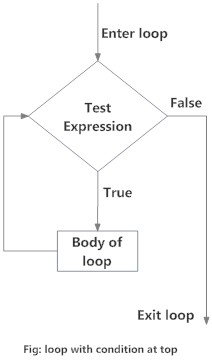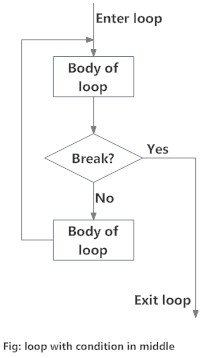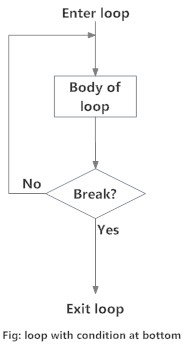Python Looping Techniques

# Python Looping Techniques

#### In this article, you'll learn to control the execution of a loop by using loop control statements like break and continue.

Python programming offers two kinds of loop, the for loop and the while loop. Using these loops along with loop control statements like break and continue, we can create various forms of loop.

## The infinite loop

We can create an infinite loop using while statement. If the condition of while loop is always `True`, we get an infinite loop.

### Example #1: Infinite loop using while

``````# An example of infinite loop
# press Ctrl + c to exit from the loop

while True:
num = int(input("Enter an integer: "))
print("The double of",num,"is",2 * num)
``````

Output

```Enter an integer: 3
The double of 3 is 6
Enter an integer: 5
The double of 5 is 10
Enter an integer: 6
The double of 6 is 12
Enter an integer:
Traceback (most recent call last):
```

## Loop with condition at the top

This is a normal while loop without break statements. The condition of the while loop is at the top and the loop terminates when this condition is `False`.

### Flowchart of Loop With Condition at Top### Example #2: Loop with condition at the top

``````
# Program to illustrate a loop with the condition at the top

# Try different numbers
n = 10

# Uncomment to get user input
#n = int(input("Enter n: "))

# initialize sum and counter
sum = 0
i = 1

while i <= n:
sum = sum + i
i = i+1    # update counter

# print the sum
print("The sum is",sum)``````

When you run the program, the output will be:

```The sum is 55
```

## Loop with condition in the middle

This kind of loop can be implemented using an infinite loop along with a conditional break in between the body of the loop.

### Flowchart of Loop with Condition in Middle### Example #3: Loop with condition in the middle

``````# Program to illustrate a loop with condition in the middle.
# Take input from the user untill a vowel is entered

vowels = "aeiouAEIOU"

# infinite loop
while True:
v = input("Enter a vowel: ")
# condition in the middle
if v in vowels:
break
print("That is not a vowel. Try again!")

print("Thank you!")
``````

Output

```Enter a vowel: r
That is not a vowel. Try again!
Enter a vowel: 6
That is not a vowel. Try again!
Enter a vowel: ,
That is not a vowel. Try again!
Enter a vowel: u
Thank you!
```

## Loop with condition at the bottom

This kind of loop ensures that the body of the loop is executed at least once. It can be implemented using an infinite loop along with a conditional break at the end. This is similar to the do...while loop in C.

### Flowchart of Loop with Condition at Bottom### Example #4: Loop with condition at the bottom

``````
# Python program to illustrate a loop with condition at the bottom
# Roll a dice untill user chooses to exit

# import random module
import random

while True:
input("Press enter to roll the dice")

# get a number between 1 to 6
num = random.randint(1,6)
print("You got",num)
option = input("Roll again?(y/n) ")

# condition
if option == 'n':
break
```
```

Output

```Press enter to roll the dice
You got 1
Roll again?(y/n) y
Press enter to roll the dice
You got 5
Roll again?(y/n) n
```